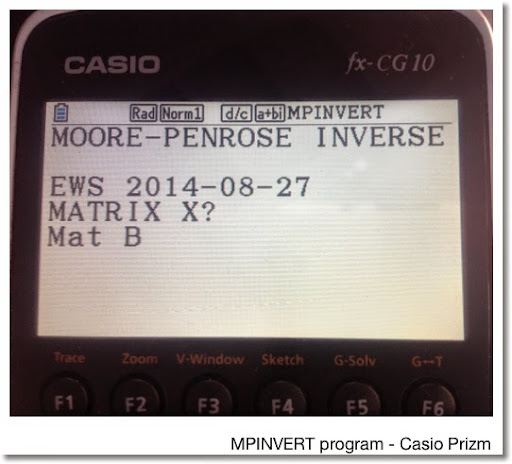## Friday, August 29, 2014

### Moore-Penrose Inverse of a MatrixMoore-Penrose Inverse of a Matrix
(E.H. Moore (1920), Arne Bjerhammar and Roger Penrose (1955))

Programs for the HP Prime and Casio Prizm (can be used for fx-9860g II and fx-9750g II) included

Also known as a pseudoinverse, the Moore-Penrose inverse of a matrix, denoted by A^+ (capital A with a supersubscript of a plus sign), is an inverse of matrix. Different from the "true" matrix inverse, the Moore-Penrose inverse allows for non-square matrices. Primarily, the Moore-Penrose inverses are calculated is assist in solving linear least-square equations.

Properties: Moore-Penrose Inverse

A A^+ A = A
A^+ A A^+ = A^+
(A A^+)^T = A
(A^+ A)^T = A^+

A^+ is unique

Calculation

One method of calculating the Moore-Penrose Inverse is the following:

For matrix A of size m × n:

Let r = row rank of A (number of linearly independent rows)
Let c = column rank of A (number of linearly independent columns)
Usually r = c
The matrix rank, denoted rank(A) = min(r, c)

If r ≤ c and rank(A) = r,
Then A^+ = A^T (A A^T)^-1

If r ≥ c and rank(A) = c,
Then A^+ = (A^T A)^-1 A^T

The programs listed on this blog entry assume the first conditions of each of the test are met, since usually, r = c.

Sources

Schott, James R. "Matrix Analysis for Statistics: 2nd Edition". Wiley & Sons. Hoboken, NJ. 2005

Wikipedia article, retrieved 8/29/2014 - URL:
http://en.m.wikipedia.org/wiki/Moore–Penrose_pseudoinverse

HP Prime Program MPINVERT

Input: MPINVERT(matrix). Execute this from Home or CAS screen.

Program:
EXPORT MPINVERT(mtx)
BEGIN
// 2014-08-27 EWS
// Moore-Penrose Matrix Inverse
LOCAL r,c,d,n;
d:=SIZE(mtx);
r:=d(1);
c:=d(2);
n:=RANK(mtx);
CASE
IF n==c THEN
RETURN (TRN(mtx)*mtx)^-1*mtx;
END;
IF n==r THEN
RETURN TRN(mtx)*(mtx*TRN(mtx))^-1;
END;
DEFAULT
RETURN "No Solution Found";
END;

END;

Casio Prizm Program MPINVERT

The program prompts for the matrix. Since the Prizm does not have a matrix rank command, it is determined by use of the rref command and pair of For loops. This program should work verbatim on the fx-9860g II and fx-9750g II.

The matrix is stored in Mat X. List 1 and Mat Y are used for calculation. The result is stored in Mat Z.

If you are using a TI-84, you would probably use an approach similar to what is presented:

Program MPINVERT
"MOORE-PENROSE INVERSE"
"EWS 2014-08-27"
"MATRIX X"? → Mat X
Dim Mat X→List 1
List 1 → R
List 1 → C
Rref Mat X → Mat Y
0 → T
For 1 → I To R
For 1 → J To C
If Mat Y[I, J] ≠ 0
Then
T + 1 → T
Break
IfEnd
Next
Next
If T = R
Then
Trn Mat X * (Mat X * Trn Mat X) ^ -1 → Mat Z
"Mat Z"
Mat Z ◢ // display
Stop
IfEnd
If T = C
Then
(Trn Mat X * Mat X) ^ -1 * Trn Mat X → Mat Z
"Mat Z"
Mat Z ◢ // display
Stop
IfEnd
"NO INVERSE"

Examples

Matrix:
[ [ 1, 2, 3 ] [ 3, 4, 0 ] ]

Moore-Penrose Inverse:
[ [ -8/229, 31/229 ] [ 6/229, 34/229 ] [ 75/229, -33/229 ] ]

Matrix:
[ [7, 4, 6, -7] [-1, 5, 3, 3] ]

Moore-Penrose Inverse: (to four decimal places)
[ [0.0489, -0.0338] [0.0194, 0.1092] [0.0360, 0.0600] [-0.0520, 0.0800] ]

This blog is property of Edward Shore. 2014

### Casio fx-CG50: Sparse Matrix Builder

Casio fx-CG50: Sparse Matrix Builder Introduction The programs can create a sparse matrix, a matrix where most of the entries have zero valu...